# Simply-supported Thick BeamThis test is used to validate the following attributes:

• Repeated eigen values

• Transverse shear effects

• Rotary inertia effects.

You will use a 1D mesh.Reference:
NAFEMS-Glasgow, Benchmark newsletter, p.16, April 1989.

##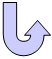Specifications

### Geometry Specifications

 Length: L = 10 m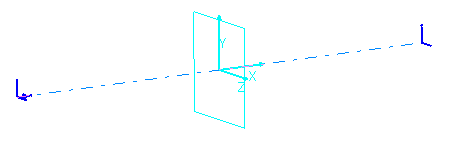Area: a = 2 m x 2 m

### Analysis Specifications

 Young Modulus (material): E = 200 MPa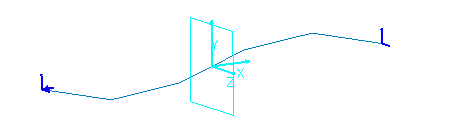Poisson's Ratio (material): ν = 0.3 Density: ρ = 8000 kg/m3 Mesh Specifications: 5 beam elements (exact 3D beam elements) Restraints (User-defined): Point A: Tx = Ty = Tz =Rx = 0 Point B: Ty = Tz = 0

##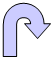Results

The results are based on the 9 lowest normal modes of the structure.
The percentages of errors are listed in the table below:

 Mode Exact [Hz] Beam [Hz] Error [%] 1 and 2 (bending modes) 42.568 42.531 0.09 3 (torsional mode) 71.5 71.554 0.08 4 (longitudinal mode) 125.51 125.515 0.00 5 and 6 (bending modes) 145.46 145.064 0.27 7 (torsional mode) 213.24 221.756 3.99 8 and 9 (bending modes) 267.01 265.79 0.46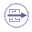To Perform the Test:

The simplysupported_thick_beam.CATAnalysis document presents a complete analysis of this case, computed with a mesh formed of beam elements.

To compute the case, proceed as follow:

1. Open the CATAnalysis document.

2. Compute the case and generate an image called Deformed mesh.